Mridul Aanjaneya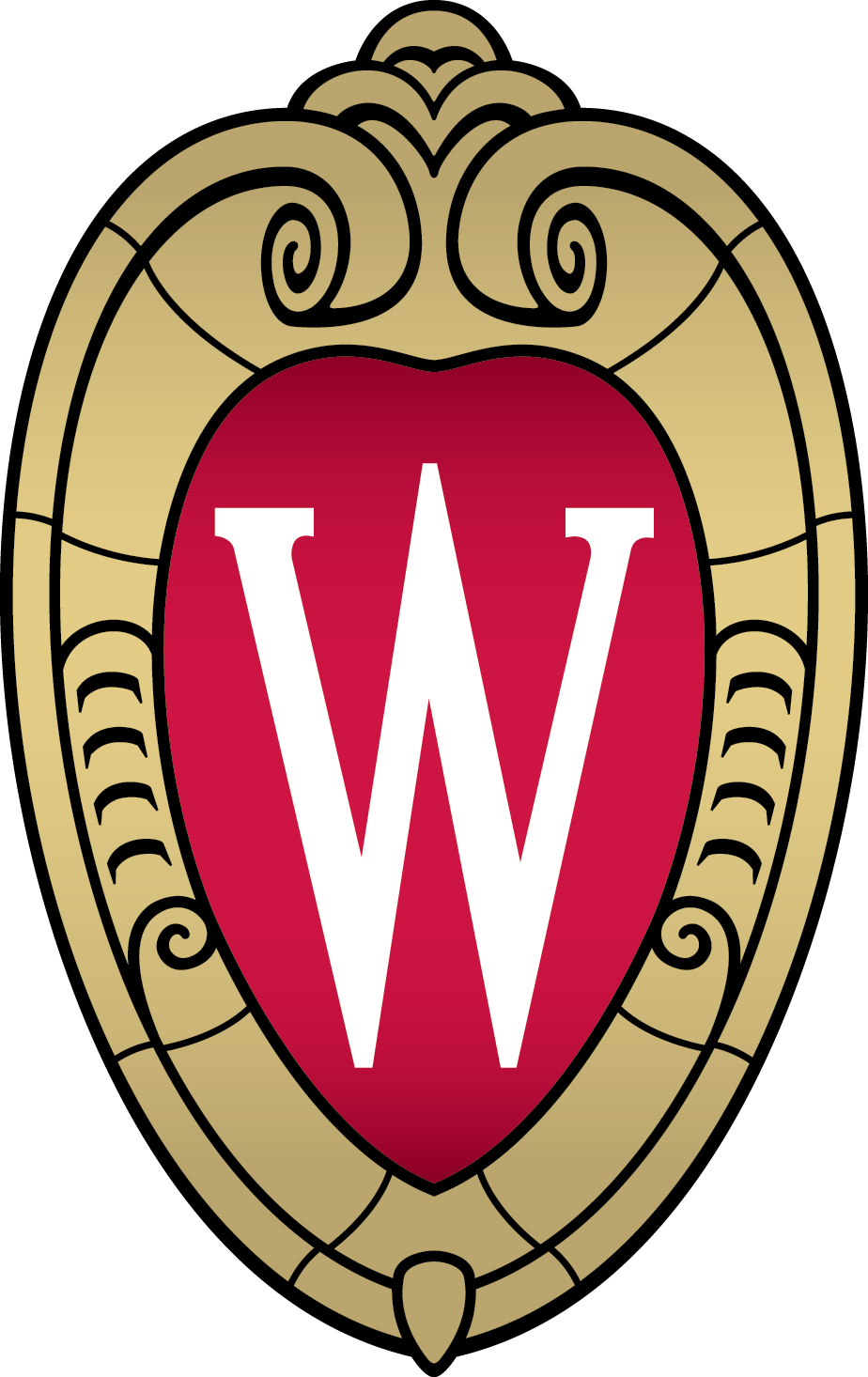Department of Computer Sciences University of Wisconsin-Madison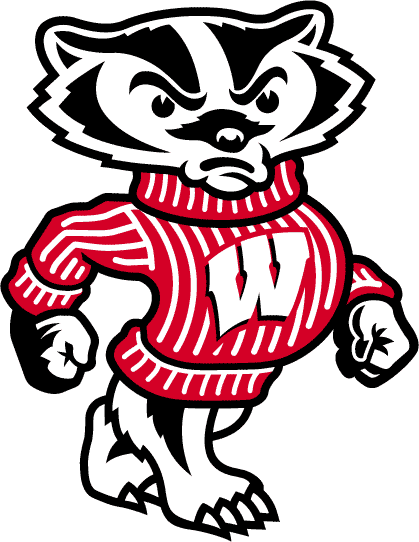## Instructor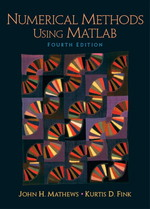CS412: Introduction to Numerical Methods University of Wisconsin - Madison (Spring 2015) Interpolation, solution of linear and nonlinear systems of equations, approximate integration and differentiation, numerical solution of ordinary differential equations. Course Website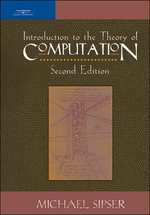CS154: Introduction to Automata Theory Stanford University (Summer 2012) Deterministic and nondeterministic finite automata, regular expressions, pumping lemma, homomorphisms, context-free languages, pushdown automata, Turing machines, decidability, NP-completeness and boolean satisfiability. Course Website

## Guest Lecturer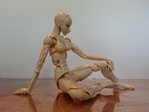CS559: Computer Graphics University of Wisconsin - Madison (Spring 2016) Lectured on homogeneous coordinates and hierarchical modeling for articulated character animation.CS838: Topics in Computing: Introduction to Physics-based Modeling and Simulation University of Wisconsin - Madison (Spring 2014) Lectured on level set methods and numerical solvers for underwater bubble simulation.

## Course Assistant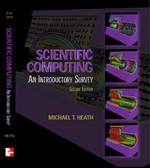CS205a: Mathematical Methods for Computer Vision, Robotics and Graphics Stanford University (Spring 2013) Continuous mathematics used in computer science (and EE) with a particular emphasis on the issues associated with designing, implementing and/or using numerical algorithms to solve equations. An underlying theme concerns the approximation issues associated with using floating-point numbers (as opposed to integers) in numerical algorithms.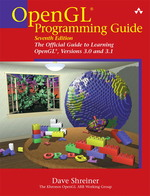CS148: Introduction to Computer Graphics Stanford University (Fall 2012) Entry-level course focusing on the technical challenges faced in capturing, displaying, and generating computer generated images. Topics include modeling of lights, cameras, materials and geometry, graphics hardware, trade-offs in physical realism and rendering time.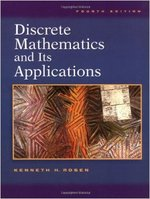CS103: Mathematical Foundations of Computing Stanford University (Spring 2010) Mathematical foundations required for computer science, including propositional predicate logic, induction, sets, functions, and relations. Formal language theory, including regular expressions, grammars, finite automata, Turing machines, and NP-completeness.